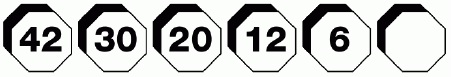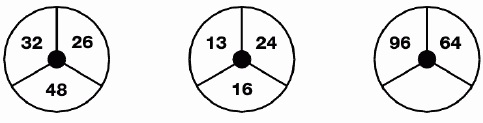## CrossWord Puzzles Questions and Answers

1. Which number will continue this sequence?Answer
 2Moving from the left to right each box contains a decreasing square number, minus the root ( 7 x 7 = 49 – 7 = 42) etc.
2. Which number is missing from the last circle?Answer
 52Divide each number in the first circle by 2 and enter the new figure one place anticlockwise in the second circle. Multiply the first set of numbers by 2 and enter this figure one place clockwise in the third circle.
12345

Page 1 of 5